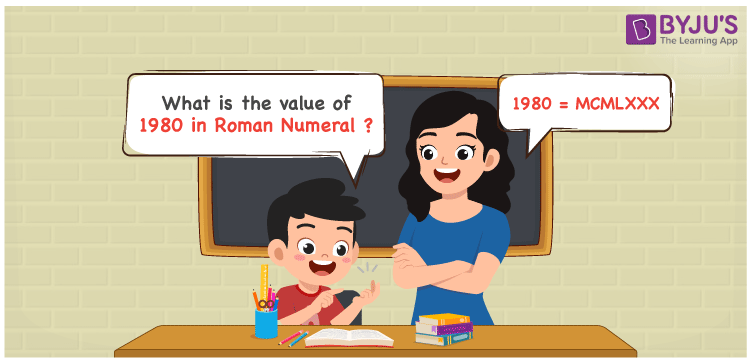# 1980 in Roman Numerals

1980 in Roman Numerals is denoted as MCMLXXX. The way of writing the numbers using the Roman alphabet is defined as Roman Numerals. The PDF format of Roman Numerals 1 to 50 helps students to grasp the Roman letters from 1 to 50 effectively. This article offers an in-depth explanation of how to represent the number 1980 in Roman Numerals.

 Number Roman Numeral 1980 MCMLXXX

## How to Write 1980 in Roman Numerals?The number 1980 in Roman Numerals can be written by following the method given below

• Break the number 1980 into the simplest form
• 1980 = 1000 + (1000 – 100) + 50 + 10 + 10 + 10
• Write their respective Roman letter and add/subtract them
• 1980 = M + (M – C) + L + X + X + X = MCMLXXX
• Thus the value of 1980 in Roman Numerals is MCMLXXX

## Video Lesson on Roman Numerals## Frequently Asked Questions on 1980 in Roman Numerals

Q1

### What is 1980 in Roman Numerals?

1980 in Roman Numerals is MCMLXXX.
Q2

### What is the value of 1000 + 980 in Roman Numerals?

1000 + 980 = 1980. The number 1980 in Roman Numerals is given by MCMLXXX. Hence the value of 1000 + 980 in Roman Numerals is MCMLXXX.
Q3

### What is the difference between 2050 and 1980 in Roman Numerals?

2050 – 1980 = 70. The number 70 in Roman Numerals is expressed as LXX. The difference between 2050 and 1980 in Roman Numerals is LXX.